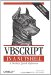# Section C.1. Arithmetic Operators

### C 1 Arithmetic Operators

+

The addition operator. Used to add numeric expressions, as well as to concatenate (join together) two string variables. However, it's preferable to use the concatenation operator with strings to eliminate ambiguity. For example:

`result = expression1 + expression2`

-

The subtraction operator. Used to find the difference between two numeric values or expressions, as well as to denote a negative value. Unlike the addition operator, it cannot be used with string variables. For example:

`result = expression1 - expression2`

/

The division operator. Returns a floating-point number.

`result = expression1 / expression2`

*

The multiplication operator. Used to multiply two numerical values. For example:

`result = expression1 * expression2`

The integer division operator. Performs division on two numeric expressions and returns an integer result (no remainder or decimal places). For example:

`result = expression1  expression2`

Mod

The modulo operator. Performs division on two numeric expressions and returns only the remainder. If either of the two numbers are floating-point numbers, they are rounded to integer values prior to the modulo operation.For example:

`result = expression1 Mod expression2`

^

The exponentiation operator. Raises a number to the power of the exponent. For example:

`result = number ^ exponent`VBScript in a Nutshell, 2nd Edition
ISBN: 0596004885
EAN: 2147483647
Year: 2003
Pages: 335